International Journal of Applied Mathematics and Theoretical Physics
Volume 3, Issue 1, January 2017, Pages: 1-6

Noninertial Freely Falling Frames Affected by Gravitational Tidal Forces

Steven D. Deines

Donatech Corporation Inc., Fairfield, Iowa, USA

Email address: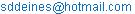To cite this article:

Steven D. Deines. Noninertial Freely Falling Frames Affected by Gravitational Tidal Forces. International Journal of Applied Mathematics and Theoretical Physics. Vol. 3, No. 1, 2017, pp. 1-6. doi: 10.11648/j.ijamtp.20170301.11

Received: August 8, 2016; Accepted: September 23, 2016; Published: November 9, 2016

Abstract: It is a major misconception that freely falling reference frames are inertial in a gravitational field. This is one outcome when employing Einstein’s principle of equivalence between a dynamical acceleration and a homogeneous gravity, which does not exist technically in nature, because gravity is radial from all mass centers, even at nearly infinite distances between masses. Even though floating objects within a space station orbiting Earth appear to move with constant inertial velocities, tidal forces exist to accelerate all such objects. The four oceanic tides of Earth prove that the Moon and Sun are the two external gravitational bodies pulling on Earth, even if an earthbound observer cannot physically feel the tidal forces. Theoretical experiments demonstrate how to observe tidal effects internally to determine the external gravitational forces. Tidal forces make dumbbell-shaped artificial satellites rotate around a heavenly body once per orbit. A liquid in free fall like mercury becomes prolate in shape and aligns with the external gravitational force if the liquid’s surface tension can be minimized. Today’s technology is very precise and can detect most subtle forces, so that local experiments can distinguish between a reference frame in free fall versus a truly inertial frame placed far away from gravitational bodies. Tidal forces always exist in any neighborhood of a test mass due to the radial gravitational force from any external mass, so the mathematical limit of a shrinking local reference frame always contains tidal forces within its domain. Thus, Einstein’s equivalence principle is an approximation and is technically applicable for only point masses.

Keywords: Freely Falling Frames, Relativity, Equivalence Principle, Local Frames

Contents

1. Introduction

In theoretical physics, a local reference frame refers to a coordinate system or frame of reference that spans a small region or domain of space (or space-time in general relativity). Local inertial frames are often synonymous with freely falling local frames in gravity. Although gravitational tidal forces can be observed, it is assumed one can have a sufficiently small, freely falling frame with an "effectively" uniform gravitational field.

The equivalence principle between gravity and dynamical acceleration was properly conceived by Einstein  in 1907, when he observed that the acceleration of bodies towards the center of the Earth with the rate of 1 g = 9.81 m/s2 at Earth’s surface was equivalent to the acceleration of a moving body observed in a rocket accelerated in free space with 1g. Einstein [2, p. 454] elaborated further on this equivalence concept. There are three forms of the equivalence principle currently used, but there are many variations between the relativity experts. For example, Weinberg [4, p. 68] wrote that "we formulate the equivalence principle as the statement that at every space-time point in an arbitrary gravitational field it is possible to choose a ‘locally inertial coordinate system’ such that, within a sufficiently small region of the point in question, the laws of nature take the same form as in unaccelerated Cartesian coordinate systems in the absence of gravitation." Weinberg admitted that how small is "sufficiently small" meant the region must be small enough so that the gravitational field is sensibly constant (approximately) throughout it. Weinberg also admitted there are references to a strong Principle of Equivalence, which applies to all laws of nature, versus the weak Principle Equivalence, which applies to all laws of motion of freely falling particles. He further attempted to distinguish between two versions of a very strong principle, which applies to all phenomena, and a medium-strong principle, which applies to all phenomena except gravitation itself. Even from one relativity expert, there are different shades of the equivalence principle.

The consensus as currently expressed in the Wikipedia lists the general guidelines for the strong, Einstein and weak equivalence principles.

Strong: (1) The outcome of any local experiment in any freely falling laboratory is independent of the velocity of the laboratory and its location in space-time, and, (2) The gravitational mass and inertial mass are identical for any object. Another variation of (2) is the gravitational motion of a small test body depends only on its initial position in space-time and velocity, and not on its constitution. (Essentially, the strong principle includes the Einstein and weak equivalence principles).

Einstein: (1) The outcome of any local experiment in any freely falling laboratory is independent of the velocity of the laboratory and its location in space-time. The Einstein equivalence encompasses the weak equivalence principles, but not necessarily the mass equivalence between inertia and gravity.

Weak: (1) The trajectory of a point mass in a gravitational field depends only on its initial position and velocity, and is independent of its composition and structure, (2) All test particles (point bodies) at the same space-time point in a gravitational field will undergo the same acceleration, independent of internal properties including rest mass, (3) All local centers of mass (effectively a point mass) will follow identical trajectories of free-fall in vacuum independent of all observable properties, (4) the local effects of motion in gravitation are indistinguishable from those of an accelerated observer without exception, and (5) the ratio of inertial mass to gravitational mass is identical for all bodies.

The two postulates of general relativity can be summarized. "All laws of nature have the same form for observers in any frame of reference, whether accelerated or not." [3, p. 1222]. "In the vicinity of any point, a gravitational field is equivalent to an accelerated frame of reference in gravity-free space (the principle of equivalence)." [3, p. 1222]. It will be shown in this paper that this last interpretation of the equivalence principle is an approximation at best.

Some of these equivalence properties can also be traced in some form from Galileo, Kepler and Newton. The real issue is how small is the freely falling laboratory required for condition (4), so that all local effects of gravitation are indistinguishable from accelerated, dynamical observations, because tidal effects from gravity do not disappear at the whim of the observer. With more precise technology available today, the tidal effects can be measured or observed in gravity with no equivalent behavior in a dynamical, accelerated laboratory in a gravity-free space. The theoretical equivalence models are only approximations that need to be updated in today’s experimental physics, because our precise technologies effectively shrink the domains of freely falling laboratories to almost a point, which negates the use of a reference frame.

2. Properties of Freely Falling Frames of Reference

According to most general relativistic applications of the equivalence principle, a freely falling coordinate system will have its equations of motion in straight geodesic lines in space-time with proper time. Weinberg [4, p. 70-73] derived the equation of motion for a freely falling system with the resulting affine connectionand the proper time differential depending on the metric tensor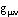. Weinberg [4, p. 73-77] showed that the derivatives of the metric tensordetermine the gravitational force in the field or affine connectionup to the first derivative. Then, Weinberg [4, p. 83-84] constructed the next simplest possible tensor with first and second derivatives of the metric tensor, which resulted in the Riemann curvature tensor. Weinberg raised the issue which equations of motion should be used by the equivalence principle for a freely falling frame. His answer was ‘scale’ determined the correct form. He defined the linear dimension of the particle be d and the gravitational field be the characteristic space-time dimension D. The ratio of the extra derivative in the Riemann curvature tensor to the affine tensor is 1/D, making the dimensional ratio roughly d/D. Weinberg concluded that unless one term in the Riemann curvature tensor is anomalously large or small, neglect the last term of this tensor if the particle is very much smaller than the gravitational field scale. He qualified that, in the case of the Moon moving in Earth’s gravitational field, the principle of equivalence must be applied to the infinitesimal elements, while the simplified equation of motion would give a fair representation. The author’s interpretation is that Weinberg demonstrates a freely falling frame is not inertial by deriving two different sets of equations of motion for the same test particle at different locations within the same reference frame. Some terms are not ignorable in different domain locations for the same reference frame, which means the freely falling frame is not inertial and not uniform for all points within the domain of the frame. Dynamical astronomy immediately shows the frame whose origin is attached to the center of mass of the freely falling Earth is dynamically accelerated. The Earth orbits around the Earth-Moon barycenter that completes an elliptical orbit about the Sun with planetary perturbations superimposed on the Earth-Moon barycenter’s orbit.

The solution of the balance equation will fix the Earth-Moon barycenter approximately ¾ of the distance from Earth’s center of mass to its surface. The balance equation describes the static torques around the barycenter and is ME RE = MM RM, where ME and MM are the respective masses of the Earth and Moon, and RE and RM are the radial distances of the Earth and Moon between the respective centers of mass and the Earth-Moon barycenter. The Earth-Moon barycenter is within the lunar orbital plane that is 5.145° from the ecliptic, as the lunar plane precesses completely in 18.6 years around this barycenter. Earth’s spin axis is 23.439° from the perpendicular to the ecliptic plane, so that the spin axis varies between 61.416° and 71.706° relative to the lunar orbital plane over 18.6 years . This does not include Earth’s own precession, nutation, or Chandler wobble of its spin axis relative to the celestial sphere (i.e. J2000 frame). The Earth-Moon barycenter has equal gravitational and centripetal forces, but this point keeps moving inside the rotating Earth. Earth’s surface experiences unmodeled torques in the standard Earth-centered, Earth-fixed frame for calculations used in inertial navigation devices, which causes approximately the same unmodeled drift diverging in the navigation calculations, regardless that different devices have advanced, more precise technologies .

The approximation of a freely falling frame as inertial is inexact, because gravitational and centripetal effects are usually unequal throughout the region of a falling frame. For example, Earth’s four ocean tides prove Earth is constantly accelerated. The force that causes the ocean tides is called tidal force. The smaller solar tide can be calculated in the same manner as the lunar tide given next. The following discussion follows Ohanian and Ruffini . Define the Earth’s center of mass as the origin of the freely falling frame. The positive z axis is the ray from the Moon’s center of mass through this origin. The distance r is the radial distance between the two centers of mass, M is Earth’s mass, and G is the gravitational constant using Newton’s law of gravity. The arbitrary x and y axes are mutually perpendicular, following the right-hand rule. A test particle on the z axis has a gravitational acceleration to the Moon of -GM/(r + z)2. The origin (z = 0) experiences a similar acceleration. The test particle’s acceleration relative to the frame’s origin is:.

where the approximation z << r is taken. A test particle on the negative z axis still has its gravitational acceleration pointing opposite to the positive z-axis, and the gravitational force relative to the origin is: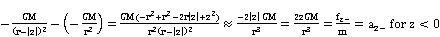.

Note that aZ+ = aZ- for the gravitational tidal acceleration, so aZ = 2z GM/r3 for the general gravitational tidal component in the z axis. The centripetal tidal force fcen points away from the Moon and is the result of Newton’s third law for an opposing force. The centripetal tidal acceleration acen in the z axis relative to the origin is: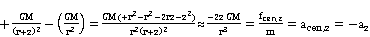.

The tidal force along the positive y axis is the gravity at the test point projected on the y axis toward the frame’s origin, as the origin has no net acceleration.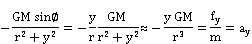where the approximation y << r is taken. Similarly, the tidal force along the positive x axis for x << r is: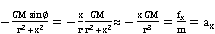.

The total tidal force due to gravity Fk or F potential on the particle in the freely falling frame is: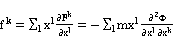.

The tidal force tensor is part of the complete Riemann curvature tensor Rmnab; and is expressed as: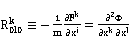.

(Since time is not included in the summation for tidal forces where dx0 = c dt, the c2 factor is not included in the tidal force tensor for simplification.) The nine quantities in Rk0l0 act on the particle located at xl by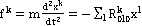where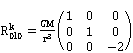.

The divergence of the tidal force fk is zero for empty space. This is easily checked.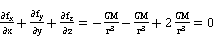.

Substitute the expression for the gravitational potential to replace the tidal forces, and Laplace’s equation Ñ2F = 0 is satisfied. To determine the tidal displacement due to the Moon on Earth’s oceans, let M be the mass of the Moon and r be the distance between the Earth and Moon. The tidal forces are:.

The potential that produces this tidal force is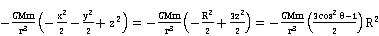.

where R = (x2 + y2+ z2)1/2 as the spherical radius of Earth and q is the angle between the radius vector and the positive z axis. For a particle m near the Earth’s surface, the particle experiences a gravitational potential due to Earth as mgh with h being the height above mean sea level and g being the local gravitational acceleration at Earth’s surface. For a unit mass m, the ocean follows the equipotential:.

The height of the ocean changes as a function of q. High tides occur at q = 0 and q = p while low tides happen at q = p/2. The range of the lunar tidal height in the open ocean is: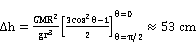(1)

It is noted that the predicted lunar tidal height at Earth’s equator (1) is nearly half of the expected and measured lunar tidal height of [8, p. 10]. The derivation (1) is based only on the existence of gravity. The term acen,z = -az was for comparison, but the centripetal terms were not in the tidal derivation. However, Newton’s third law requires that the centripetal force exists simultaneously with gravity as Earth orbits about the mutual barycenters between the Earth and Moon and between the Earth and Sun. The centripetal force effectively pulls the solid Earth away from the Moon, while the ocean is pulled toward the Moon, which effectively doubles the tidal height predicted by Equation (1). Since (1) is an approximation to two significant figures, use Einstein’s equivalence principle to replace the centripetal force as an equivalent gravity in the opposite direction, which will double the prediction of (1). Ohanian and Ruffini’s result  would now be 2 x 0.53 cm = 1.06 m, which compares closely to Olah’s lunar tidal height of 1.1 m [8, p. 10].

To confine a test for the tidal force to a small laboratory orbiting Earth, consider measuring the ratio Dh/R where M is now the mass of Earth, r is the distance between Earth’s center of mass and the orbiting space station (a circular orbit would make r constant), R is the radius of a large mass of mercury, and g is the acceleration of gravity from the mercury at its own surface. If a large quantity of mercury is used, the surface tension is reduced compared to the tidal forces that the mercury mass will experience from Earth’s gravity. For the sake of argument, the surface tension of mercury is ignored. The gravity from the mercury liquid can be written as g = G(4pr R3/3)/R2 with r being the density of mercury. Then,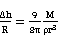(2)

The remarkable feature of (2) is that the shape of the mercury test mass is independent of its size R. The prolateness of the tidal ellipsoid of the deformed mercury test fluid is a local measure of the external gravitational force. Even though the expressions for tidal forces are valid only for small displacements, the tidal lines of force can be drawn showing the converging lines point to the gravitational source, which is Earth’s center of mass in this case. There are more practical techniques that can detect the local gravity within a freely falling frame, such as detecting torques with rigid rods.

Artificial satellites are affected by gravity and centripetal forces that create torques. Gravity gradient stabilization has been demonstrated very successfully for artificial satellites that have relaxed accuracy requirements to point antennas passively toward the Earth. The principle can be easily understood by the model of a dumbbell satellite consisting of two identical masses and connected by a rod of uniform density of length 2R. A deflection away from the local vertical generates two restoring torques that each is caused by the imbalance of gravitational and centripetal forces acting on the two masses. Suppose the two end masses are located R from the satellite’s center of mass and have an angular displacement clockwise of the local vertical. The centripetal force, Cf, on the farther mass is greater than the gravitational force, g, on it, and the gravitational force on the closer mass, G, is greater than its centripetal force, cf. Two combined torques are generated to force each mass to the local vertical relative to Earth as R x G + (R x -cf) + (-R x g) + (-R x –Cf) = R x (G - g) + R x (Cf – cf) by the right hand rule (or counterclockwise). Kaplan [9, p. 200-205] derived the mathematics, which also incorporated Euler’s equations, that showed the stabilizing torques were produced when either of two conditions of a satellite’s construction were met: Ipitch > Iroll > Iyaw or Iroll > Iyaw > Ipitch. Of these, the former solution has a much larger region of stability. This gravity gradient effect in any local frame has been demonstrated repeatedly with artificial satellites to cause the satellite body to rotate synchronously each time it orbits around Earth. This passive body rotation of a rigid rod orbiting a heavenly body demonstrates the freely falling frame is noninertial.

This scale can be shrunk arbitrarily. The quantum limit of a rigid rod is a diatomic molecule (e.g. oxygen, hydrogen, chlorine, etc.) at its lowest energy state as a torque detector of gravity. However, interfering electromagnetic interaction of other diatomic molecules, besides the kinetic collisions, may make this test molecule impractical. Theoretically, one can replace the dumbbell body with two test masses connected by a massless rod of length L. In the limit as the length L approaches zero, the gravity gradient and coexisting centripetal gradient always exist in any theoretical neighborhood of radius L/2. This means that any local frame orbiting another body is never small enough to guarantee gravity is effectively homogeneous, so inhomogeneous effects, like tides, are always present in all mathematical limits of any shrinking neighborhood, regardless of the macroscopic size or scale of the local falling frame. Thus, any falling frame in a gravity gradient is noninertial. The center of mass of an orbiting body experiences no net forces, but all other points within the domain of the falling reference frame will experience tidal torques, even if the freely falling body is not rotating initially.

To emphasize this point even further, consider an astronaut inside a hollow sphere of uniform density, r, orbiting Earth with no other gravitational bodies in this scenario. The gravitational potential F has been solved for the regions outside of the sphere of radius a, within the shell itself, and inside the hollow cavity of radius b [10, p. 82]. Inside the cavity where R < b, F = -2pGr(a2-b2), which is constant. The gravitational force due to this hollow sphere is fg = -dF/dR = 0 for an astronaut inside the cavity. But, gravitational potentials are additive, and Earth’s potential FE at the astronaut’s location contributes a total potential of F + FE, so that f = -d(F + FE)/dR ¹ 0 inside the cavity. If the hollow sphere was conductive, then the internal cavity would be electrically neutral and shielded from all external electrical fields. A conductive, hollow sphere can shield the cavity from external electromagnetic fields, but fails to shield the cavity from external gravitational fields. This dichotomy is a critical test case that any Grand Unification Theory has failed to resolve and is an excellent test for the ongoing string theory development. Inside the cavity, the astronaut can let multiple rods with masses concentrated on the rod ends oscillate about the local vertical vectors from Earth. Ignoring the practical issues of measuring the directional angles and the gravitational attraction between the rods and the astronaut (or remove the astronaut from the orbiting sphere), the rods would oscillate and point to a common convergence point. The geometry of the angles (i.e. parallax) and the separation between the individual rods would indicate how far away Earth’s center of gravity was from the orbiting hollow sphere. Even without such tools, the astronaut’s own hair could theoretically sense the difference between a centripetal pull with splayed (diverging) hair and gravitational pull with converging hair, provided the nerves attached to the hair follicles were sufficiently sensitive and the hairs were flexible and long enough.

Einstein  approximated gravity by requiring an external, static, homogeneous gravitational field. His example equates homogeneous gravity pulling on a stationary enclosed box to a box with no gravity that is pulled by a cable with constant acceleration in the opposite direction. This concept also requires the ratio of inertial masses to gravitational masses to be an identical ratio for all bodies (which is outside the scope of this paper) as verified by the many Eötvös torsion balance experiments with no detectable differences [4, Chapter 1], which all indicate a ratio of exactly one. Homogeneous gravity does not exist in the universe, because gravitational potentials emanate from centers of masses, are additive, and cannot be shielded from other external gravitational sources. Even in the unrealistic conditions of a hollow sphere of uniform density, an observer inside the cavity still detects external gravitational forces through tidal force measurements.

Ohanian and Ruffini [7, p. 53] wrote "Local experiments can distinguish between a reference frame in free fall in a gravitational field and a truly inertial reference frame placed far away from all gravitational fields. Local experiments can distinguish between a reference frame at rest in a gravitational field and an accelerated reference frame far away from all gravitational fields. Gravitational effects are not equivalent to the effects arising from an observer’s acceleration." Summarizing, only the center of mass of a freely falling body has no net torque, but all other points will experience tidal forces, because they are displaced a physical distance from that center of gravity. A domain of a freely falling frame with its origin attached to the center of gravity of a falling mass is noninertial and accelerated at all points by unequal tidal forces (except for the solitary origin), regardless if the frame is not rotating.

3. Conclusion

The concepts behind the weak, Einstein and strong equivalence principle between gravitational and dynamical accelerations are examined. According to the consensus expressed in the Wikipedia, the strong principle assumes that gravitational and inertial masses are identical and incorporates Einstein equivalence. The Einstein equivalence principle assumes the ratio between gravitational and inertial mass is fixed for all bodies and all outcomes of any local nongravitated experiment of a freely falling laboratory is independent of the velocity of the laboratory and its location in the gravity field, while including all concepts within weak equivalence principles. The weak equivalence principles are based on point masses and the trajectories executed in the presence of gravity, which is true, except for the concept that the local effects of motion within gravity are indistinguishable from those same local effects witnessed by an observer in a dynamical laboratory given the same acceleration without gravity. It is demonstrated that gravitational tidal effects are always present in any local freely falling laboratory in gravity. Earth has four tides caused by the Moon and Sun, which each create a pair of tides from gravity and the associated centripetal acceleration that is required by Newton’s third law. A freely falling liquid such as mercury will be prolate in shape and point toward any external gravity, no matter the size of the liquid if surface tension can be minimized. The passive artificial satellites shaped with a dumbbell mass distribution will rotate once every orbital period by pointing constantly toward Earth, which is easily achieved with a length of a few meters. Even if the observer is shielded by a hollow sphere of uniform density to produce no internal gravity, any external gravity will still be detected since gravitational potentials are always additive everywhere. It is interesting to note that if the hollow sphere was conductive, the hollow sphere would shield the observer inside from external electromagnetic forces, but not gravity. This example is one major reason that unified field theories have failed to resolve, and it is an excellent test for the ongoing string theory development. Einstein’s equivalence principle is based on exchanging any dynamical acceleration with a homogeneous gravity, which does not exist in nature. As all gravity fields emanate radially from the centers of mass of every object, Einstein’s equivalence is an approximation in actual practice and is only technically correct when dealing with point masses. Thus, any freely falling reference frame that has finite three-dimensional coordinates defining the domain of space within the frame will be noninertial in practice.

References

1. Einstein, A., "How I Constructed the Theory of Relativity", translated by Masahiro Morikawa from the text recorded in Japanese by Jun Ishiwara, Association of Asia Pacific Physical Societies (AAPPS) Bulletin, Vol. 15, No. 2, pp. 17-19, April 2005. Einstein recalled events of 1907 in a talk in Japan on 14 December 1922.
2. Einstein, A., "Relativitätsprinzip und die aus demselben gezogenen Folgerungen (On the Relativity Principle and the Conclusions Drawn from It)", Jahrbuch der Radioaktivität (Yearbook of Radioactivity), (1908) 4: pp. 411–462.
3. Serway, R. A. and Jewett Jr., J. W., Physics for Scientists and Engineers, Cengage Learning, 9th ed. (2014).
4. Weinberg, S., Gravitation and Cosmology: Principles and Applications of the General Theory of Relativity, John Wiley & Sons, New York (1972).
5. Seidelmann, P. K., [Ed.] Explanatory Supplement to the Astronomical Almanac, U.S. Naval Observatory, University Science Books (1992).
6. Britting, K. R., Inertial Navigation Systems Analysis, Wiley & Sons (1971).
7. Ohanian, H. C. and Ruffini, R., Gravitation and Spacetime, 2nd ed., Norton and Co., New York (1994).
8. Olah, S., "Solar and Lunar Tides", General Physics Journal (2009).
9. Kaplan, M. H., Modern Spacecraft Dynamics and Control, John Wiley & Sons, New York (1976).
10. Marion, J. B., Classical Dynamics of Particles and Systems, 2nd ed., Academic Press, New York (1970).
11. Einstein, A., "The effect of gravity on light", (1911), translated and reprinted in The Principle of Relativity, by Davis, F. A., Dover Publications (1928).

 Contents 1. 2. 3.
Article ToolsAbstractPDF(191K)
Follow on us
PUBLICATION SERVICE
JOIN US
RESOURCES
SPECIAL SERVICES
ADDRESS
Science Publishing Group
548 FASHION AVENUE
NEW YORK, NY 10018
U.S.A.
Tel: (001)347-688-8931